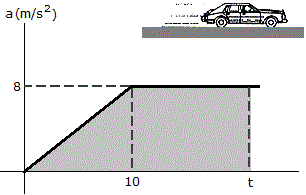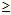# Engineering Mechanics - Kinematics of Particle (KOP) - Discussion

### Discussion :: Kinematics of Particle (KOP) - General Questions (Q.No.1)

1.A race car starting from rest moves along a straight track with an acceleration as shown in the graph (where for t10 s, a = 8 m/s2). Determine the time t for the car to reach a speed of 50 m/s.

 [A]. t = 11.25 s [B]. t = 6.25 s [C]. t = 12.5 s [D]. t = 3.53 s

Explanation:

No answer description available for this question.

 Abhishek Chouksey said: (Apr 15, 2011) Its Simple> in first 10 sec it reaches only 40 m/s=1/2*10*8(uniform acc) in next 1.25 sec at constant acc.(v=u+at) it tends to 50 m/s.

 Puru said: (Jun 3, 2011) Dear, please tell me how it comes 40m/s. By which law and logic you calculate 40m/s. FYI 1. V=u+at 3. v2=u2+2as 2. s=ut+1/2at2 4. s=1/2(v+u)t

 Gopala Krishna said: (Jun 30, 2011) Here the car is not moving with uniform accleration upto ten seconds area of the triangle=0.5*8*10=40m/sec(t=10) now accleration is constant So apply v=u+at 50=40+8*t t=1.25 So total timet = 10+1.25 = 11.25

 Mani said: (Aug 25, 2011) S=1/2 (8+0) 10. Since initial velocity is zero. My guess.

 Santhosha said: (Aug 31, 2011) Initially the is at rest, we consider u=40m/s, and v=50m/s then v=u+at 50=40+8*t t=1.25sec, This is the time required to travel from 40m/s velocity to 50m/s velocity. then the time required to travel the car from rest position is 10+1.25=11.25 sec.

 Ankit Bhardwaj said: (Sep 24, 2011) That's very simple... We know dat a= dv/dt or we can say that v= integral(a dt)...............(1) Now by graph we can see that a=(4/5)t upto the first 10 seconds Now put v=50 in the eq.(1) So after calculating t= 11.25 sec.

 Sekhar said: (Sep 15, 2012) Actually we want calculate and differentiating function, like ankith what he was explain.

 Wasprulez1990 said: (Oct 7, 2012) The area in the graph is velocity i.e. v=at, so the total area is given i.e.final velocity 50 m/s, So 50= area of triangle + area of rectangle. 50= (0.5 x base x height) + (width x height). 50= (0.5 x 10 x 8) + [ (t-10) x 8]. Finally t=11.25 seconds.

 Aishwarya Katale said: (Dec 2, 2013) How to calculate 40? which is v?

 Ankit Kumar said: (Oct 27, 2014) Aishwarya Katale : Actually 40m/s its area under the curve. :-).

 Manan said: (Dec 7, 2014) The answer is incorrect correct answer is 6.25s. Solution u = 0. v = 50 m/s. a = 8 m/s^2. So just find t by v = u+at. Which is 50 = 0+8t? 50 = 8t. t = 50\8 = 6.25s.

 Hasya said: (May 17, 2016) No, 11.25 is correct. The formula v = u + at is applicable only in the case of constant acceleration. Since up to the first 10 seconds the car is moving wth increasing acceleration area under the triangle gives final velocity at t =10 sec since initial velocity is 0.

 Kumara Swamy said: (May 31, 2016) 11.25 secs are the correct answer. Equations of motion are: v = u + at;. s = ut + 0. 5 at^2 etc are valid when accleration is constant. In the question, the acceleration varies with timeup to 10 sec and then it is constant so, use v = u + at after 10 secs. And for before 10 secs use an area under a - t graph is the velocity similarly area under v - t graph gives displacement. You will understand this concept when you pass through basics of calculus.

 Srikanth said: (Aug 10, 2016) Acceleration * time = velocity. So,in the above graph find the area covered by line up to uniform acceleration. i.e., Area of triangle = 1/2 * 10 * 8 = 40 m/s. And then it follows constant acceleration and the required area(rectangle) is 10. So 8 * 1.25 = 10 m/s, so a total of (40 + 10)m/s is achieved in (10 + 1.25)secs.

 Ss Ahamed Halith said: (Oct 10, 2019) 1. We consider the given acceleration and time (i.e a=8 ms-2 t=10s) then we find velocity for that next we pick any one choices given and add or sub for making it equal to 10 find another velocity.